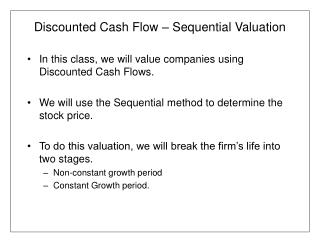DownloadDownload PresentationDiscounted Cash Flow – Sequential Valuation

# Discounted Cash Flow – Sequential Valuation

Download Presentation## Discounted Cash Flow – Sequential Valuation

- - - - - - - - - - - - - - - - - - - - - - - - - - - E N D - - - - - - - - - - - - - - - - - - - - - - - - - - -
##### Presentation Transcript

1. Discounted Cash Flow – Sequential Valuation • In this class, we will value companies using Discounted Cash Flows. • We will use the Sequential method to determine the stock price. • To do this valuation, we will break the firm’s life into two stages. • Non-constant growth period • Constant Growth period.

2. Discounted Cash Flow – Sequential Valuation • To value the cash flows during the non-constant growth period, we need • Estimate Cash flow for all years • Discount cash flow • Note: growth annuity calculation may be helpful but can only use if r > g! • During the non-constant growth period, the firm may go through many different growth cycles. • Only when the we can assume that growth is stable and will remain unchanged, does the non-constant growth period end.

3. Discounted Cash Flow – Sequential Valuation • Once the non-constant growth ends, the constant growth period begins. • To value the firm during the constant growth period, we must calculate a Terminal Value • Two ways typically calculated • If can estimate constant growth rate, use perpetuity equation. • Timing is essential here. • You use the cash flow after one year of the constant growth rate. • This gives the value at the point when growth became constant, so the value must be discounted to t=0. • If you are unable to estimate constant growth, you can use an exit multiple.

4. Three Rules for Valuing Cash flows • Different cashflows have different levels. • A firm could have different divisions with different risk levels. • Certain cashflows could be certain and others are uncertain or risk. • Example: If you know you will have \$20,000 of depreciation for the next 10 years and the tax rate is 30%, then the depreciation tax shield is a riskless cash flow • Use risk-adjusted discount rates to value risky cash flows • Use the risk-free discount rate to value riskless cashflow

5. Three Rules for Valuing Cash flows • Be consistent in your treatment of inflation • Use nominal cash flows and a discount rate that accounts for inflation. • Use real cash flows and a discount rate that ignores the effect of inflation. • Consider the timing of the cash flows • End of the year versus Beginning of the year. • Best approximation: Mid-year discounting.

6. Valuation Example: Motel • From the Benninga and Sarig text book, Chapter 3, Cash Flow this year is • Profit After Tax (net income)- 30,203 • Depreciation - 20,000 • Interest Expense (net of tax) 22,496 • Free Cash Flow 72,699 • Change Assumptions from the book • FCF (excluding depreciation tax shield) grows at 10% for the next 10 years (the book assumed it was constant). This is above the industry average. • After 10 years, growth stabilizes. However, you are uncertain what the stable growth rate will be. • You know that similar real estate assets have a price to earnings ratio of 8 and you expect the same for your hotel in 10 years.

7. Valuation Example: Motel • Different Cash flows have different risks. So, when you value the cash flow consider different risk levels. • Free Cash Flow 72,699 • Depreciation Tax Shield (20,000 x .30304) • Operating Cash Flow (residual)

8. Cashflow and Inflation Adjustments • Is the depreciation tax shield a real or nominal cashflow? • It will not change so it is nominal , so it must be discounted using an rate including inflation (nominal rate) • Is it a risky cash flow? • No, so we use the nominal, riskfree rate. • Is the cash flow for operations a real or nominal cashflow? • Our estimates do not account for changes that occur due to inflation, so it is a real cash flow and must be discounted using a real rate. • Is it a risky cash flow? • Yes, so we use the real, risky rate. • Both of these cashflow accrue throughout the year, so they are discounted at mid-year.

9. Cashflow and Inflation Adjustments • The terminal value • Is the terminal cashflow nominal or real? • If it is based on nominal earnings, then it is nominal • Is it risky? – yes, • so nominal risky rate is used. • Terminal Value using exit multiple

10. Discount Rate • Real RADR (risky) = 20% (given) • Real Riskfree = 7% (given) • Inflation = 3% (given) • Nominal RADR = • Nominal Riskfree =

11. Valuing the Firm • PVA (real operating CF, g=10%, 20%, 10 yrs at mid-year) • PVA (nominal tax shield CF, 10.21%, 10 yrs at mid-year) • PV (Terminal Value, 10 yrs, 23.6%) • Firm Value • Equity Value • Debt value given in text.